# What is Decision Tree in Data Mining? Types, Real World Examples & Applications

## Introduction to Data Mining

Data is often present as the raw data which needs to be effectively processed for converting it into useful information. The prediction of the outcomes often relies on the process of finding patterns, anomalies, or correlations within the data. The process was termed the “knowledge discovery in databases”.

It was only in the 1990s when the term “data mining” was coined. Data mining was founded over three disciplines: statistics, artificial intelligence, and machine learning.  Automated data mining has shifted the process of analysis from a tedious to a faster approach. Data mining allows the user to

• Remove all the noisy and chaotic data
• Understand the relevant data and use it for the prediction of useful information.
• The process of predicting informed decisions is accelerated.

Data mining might also be referred to as the process of identifying hidden patterns of information which require categorization. Only then the data can be converted into useful data. The useful data can be fed into a data warehouse, data mining algorithms, data analysis for decision making.

## Decision tree in Data mining

A type of data mining technique, Decision tree in data mining builds a model for classification of data. The models are built in the form of the tree structure and hence belong to the supervised form of learning. Other than the classification models, decision trees are used for building regression models for predicting class labels or values aiding the decision-making process. Both the numerical and categorical data like gender, age, etc. can be used by a decision tree.

### Structure of a decision tree

The structure of a decision tree consists of a root node, branches, and leaf nodes. The branched nodes are the outcomes of a tree and the internal nodes represent the test on an attribute. The leaf nodes represent a class label.

### Working of a decision tree

1. A decision tree works under the supervised learning approach for both discreet and continuous variables. The dataset is split into subsets on the basis of the dataset’s most significant attribute. Identification of the attribute and splitting is done through the algorithms.

2. The structure of the decision tree consists of the root node, which is the significant predictor node. The process of splitting occurs from the decision nodes which are the sub-nodes of the tree. The nodes which do not split further are termed as the leaf or terminal nodes.

3. The dataset is divided into homogenous and non-overlapping regions following a top-down approach. The top layer provides the observations at a single place which then splits into branches. The process is termed as “Greedy Approach” due to its focus only on the current node rather than the future nodes.

4. Until and unless a stop criterion is reached, the decision tree will keep on running.

5. With the building of a decision tree, lots of noise and outliers are generated. To remove these outliers and noisy data, a method of “Tree pruning” is applied. Hence, the accuracy of the model increases.

6. Accuracy of a model is checked on a test set consisting of test tuples and class labels. An accurate model is defined based on the percentages of classification test set tuples and classes by the model.

Figure 1: An example of an unpruned and a pruned tree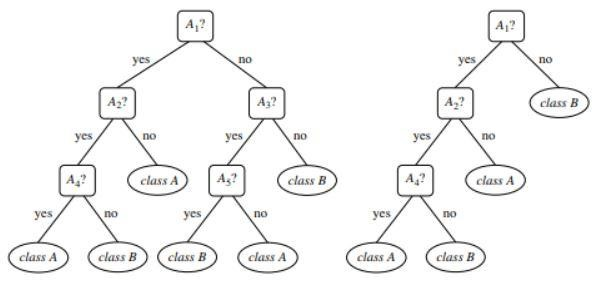Source

## Types of Decision Tree

Decision trees lead to the development of models for classification and regression based on a tree-like structure. The data is broken down into smaller subsets. The result of a decision tree is a tree with decision nodes and leaf nodes. Two types of decision trees are explained below:

### 1. Classification

The classification includes the building up of models describing important class labels. They are applied in the areas of machine learning and pattern recognition. Decision trees in machine learning through classification models lead to Fraud detection, medical diagnosis, etc. Two step process of a classification model includes:

• Learning: A classification model based on the training data is built.
• Classification: Model accuracy is checked and then used for classification of the new data. Class labels are in the form of discrete values like “yes”, or “no”, etc.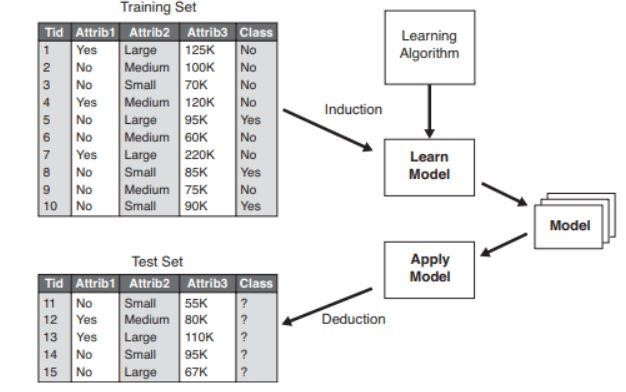Figure 2: Example of a classification model.

### 2. Regression

Regression models are used for the regression analysis of data, i.e. the prediction of numerical attributes.  These are also called continuous values. Therefore, instead of predicting the class labels, the regression model predicts the continuous values.

List of Algorithms Used

A decision tree algorithm known as “ID3” was developed in 1980 by a machine researcher named, J. Ross Quinlan. This algorithm was succeeded by other algorithms like C4.5 developed by him. Both the algorithms applied the greedy approach. The algorithm C4.5 doesn’t use backtracking and the trees are constructed in a top-down recursive divide and conquer manner. The algorithm used a training dataset with class labels which get divided into smaller subsets as the tree gets constructed.

• Three parameters are selected initially- attribute list, attribute selection method, and data partition. Attributes of the training set are described in the attribute list.
• Attribution selection method includes the method for selection of the best attribute for discrimination among the tuples.
• A tree structure depends on the attribute selection method.
• The construction of a tree starts with a single node.
• Splitting of the tuples occurs when different class labels are represented in a tuple. This will lead to the branch formation of the tree.
• The method of splitting determines which attribute should be selected for the data partition. Based on this method, the branches are grown from a node based on the outcome of the test.
• The method of splitting and partitioning is recursively carried out, ultimately resulting in a decision tree for the training dataset tuples.
• The process of tree formation keeps on going until and unless the tuples left cannot be partitioned further.
• The complexity of the algorithm is denoted by

n * |D| * log |D|

Where, n is the number of attributes in training dataset D and |D| is the number of tuples.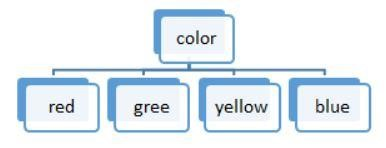Source

Figure 3: A discrete value splitting

The lists of algorithms used in a decision tree are:

ID3

The whole set of data S is considered as the root node while forming the decision tree. Iteration is then carried out on every attribute and splitting of the data into fragments. The algorithm checks and takes those attributes which were not taken before the iterated ones. Splitting data in the ID3 algorithm is time consuming and is not an ideal algorithm as it overfits the data.

C4.5

It is an advanced form of an algorithm as the data are classified as samples. Both continuous and discrete values can be handled efficiently unlike ID3. Method of pruning is present which removes the unwanted branches.

CART

Both classification and regression tasks can be performed by the algorithm. Unlike ID3 and C4.5, decision points are created by considering the Gini index. A greedy algorithm is applied for the splitting method aiming to reduce the cost function. In classification tasks, the Gini index is used as the cost function to indicate the purity of leaf nodes. In regression tasks, sum squared error is used as the cost function to find the best prediction.

CHAID

As the name suggests, it stands for Chi-square Automatic Interaction Detector, a process dealing with any type of variables. They might be nominal, ordinal, or continuous variables. Regression trees use the F-test, while the Chi-square test is used in the classification model.

MARS

It stands for Multivariate adaptive regression splines. The algorithm is specially implemented in regression tasks, where the data is mostly non-linear.

Greedy Recursive Binary Splitting

A binary splitting method occurs resulting in two branches. Splitting of the tuples is carried out with the calculation of the split cost function. The lowest cost split is selected and the process is recursively carried out to calculate the cost function of the other tuples.

## Decision Tree with Real World Example

Predict loan eligibility process from given data.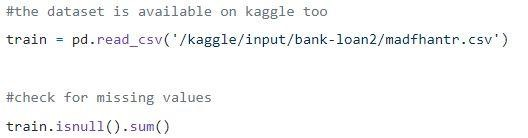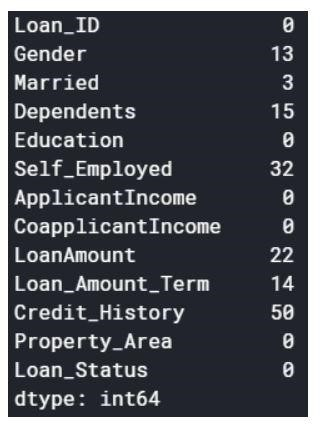The null values can be either dropped off or filled with some values. The original dataset’s shape was (614,13), and the new data-set after dropping the null values is (480,13).

Step2: a look at the dataset.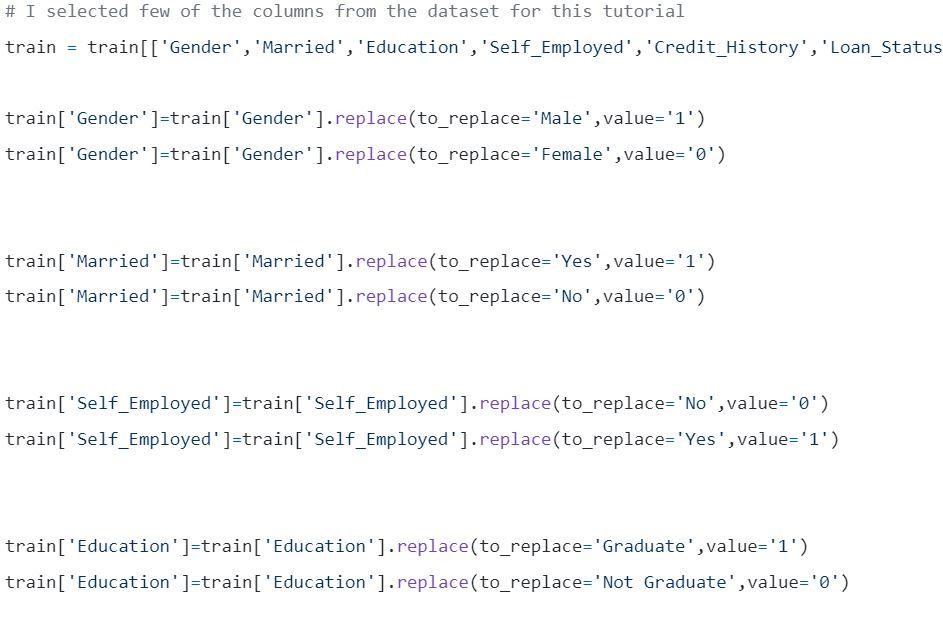Step3: Splitting the data into training and test sets.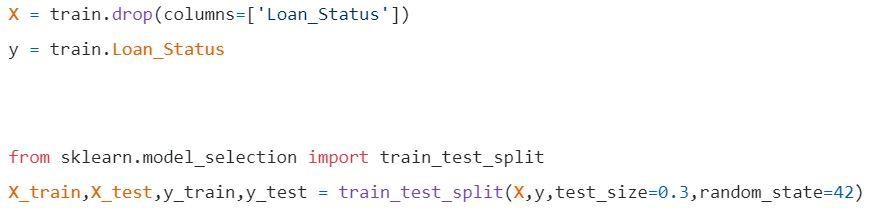Step 4: Build the model and fit the train set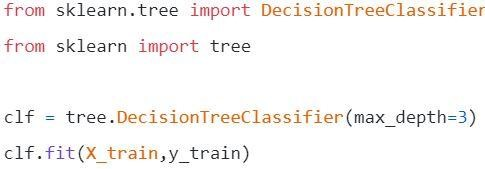Before visualization some calculations are to be made.

Calculation 1: calculate the entropy of the total dataset.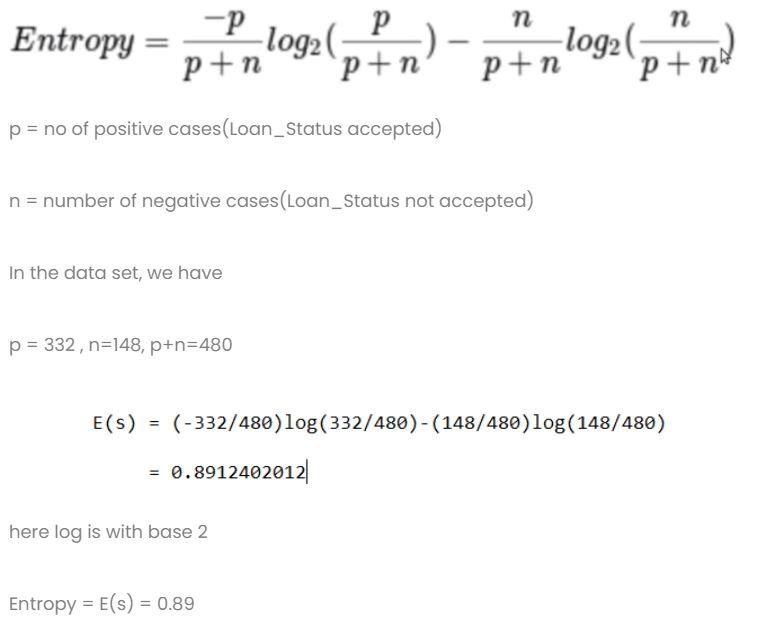Calculation 2: Find the entropy and gain for every column.

1. Gender column
• Condition 1: data-set with all male’s in it and then,

p = 278, n=116 , p+n=489

Entropy(G=Male) = 0.87

• Condition 2: data-set with all female’s in it and then,

p = 54 , n = 32 , p+n = 86

Entropy(G=Female) = 0.95

• Average information in gender column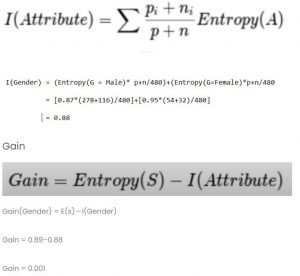1. Married column
• Condition 1: Married = Yes(1)

In this split the whole data-set with Married status yes

p = 227 , n = 84 , p+n = 311

E(Married = Yes) = 0.84

• Condition 2: Married = No(0)

In this split the whole data-set with Married status no

p = 105 , n = 64 , p+n = 169

E(Married = No) = 0.957

• Average Information in Married column is
1. Educational column
• Condition 1: Education = Graduate(1)

p = 271 , n = 112 , p+n = 383

• Condition 2: Education = Not Graduate(0)

p = 61 , n = 36 , p+n = 97

E(Education = Not Graduate) = 0.95

• Average Information of Education column= 0.886

Gain = 0.01

4) Self-Employed Column

• Condition 1: Self-Employed = Yes(1)

p = 43 , n = 23 , p+n = 66

E(Self-Employed=Yes) = 0.93

• Condition 2: Self-Employed = No(0)

p = 289 , n = 125 , p+n = 414

E(Self-Employed=No) = 0.88

• Average Information in Self-Employed in Education Column = 0.886

Gain = 0.01

1. Credit Score column: the column has 0 and 1 value.
• Condition 1: Credit Score = 1

p = 325 , n = 85 , p+n = 410

E(Credit Score = 1) = 0.73

• Condition 2: Credit Score = 0

p = 63 , n = 7 , p+n = 70

E(Credit Score = 0) = 0.46

• Average Information in Credit Score column = 0.69

Gain = 0.2

Compare all the gain values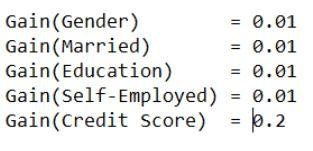Credit score has the highest gain. Hence, it will be used as the root node.

Step 5: Visualize the Decision Tree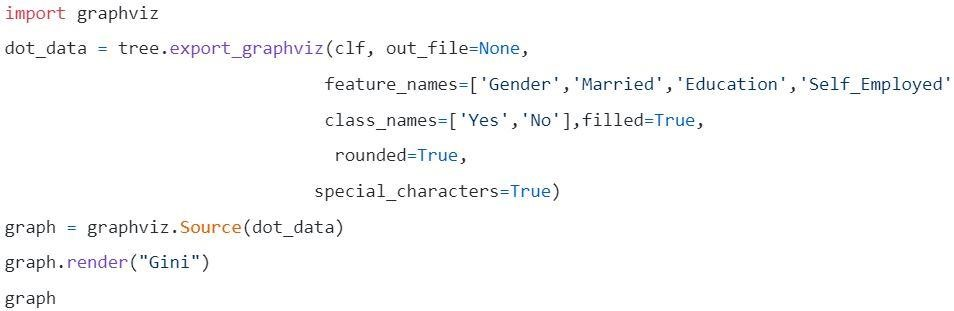Figure 5: Decision tree with criterion Gini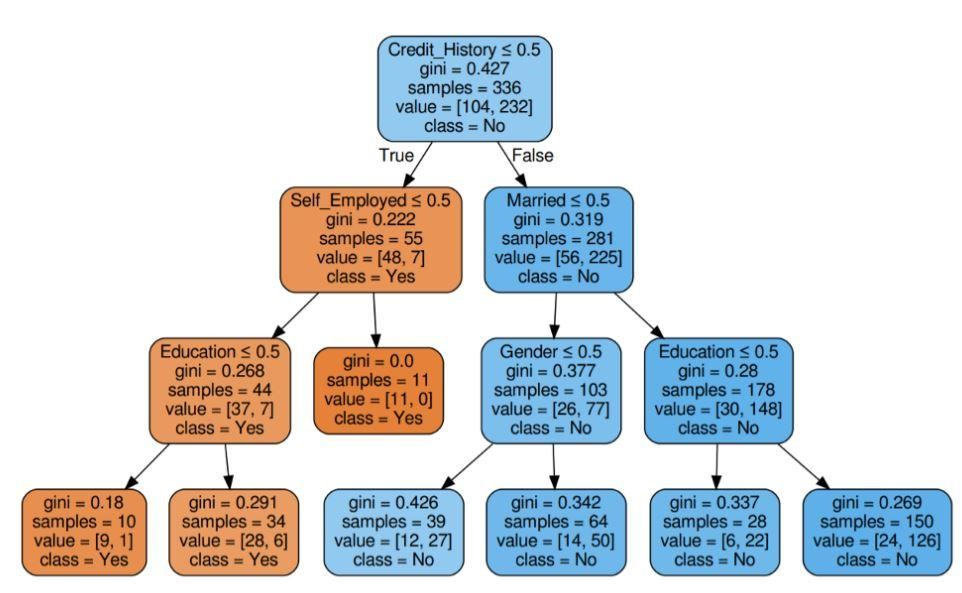Source

Figure 6: Decision tree with criterion entropy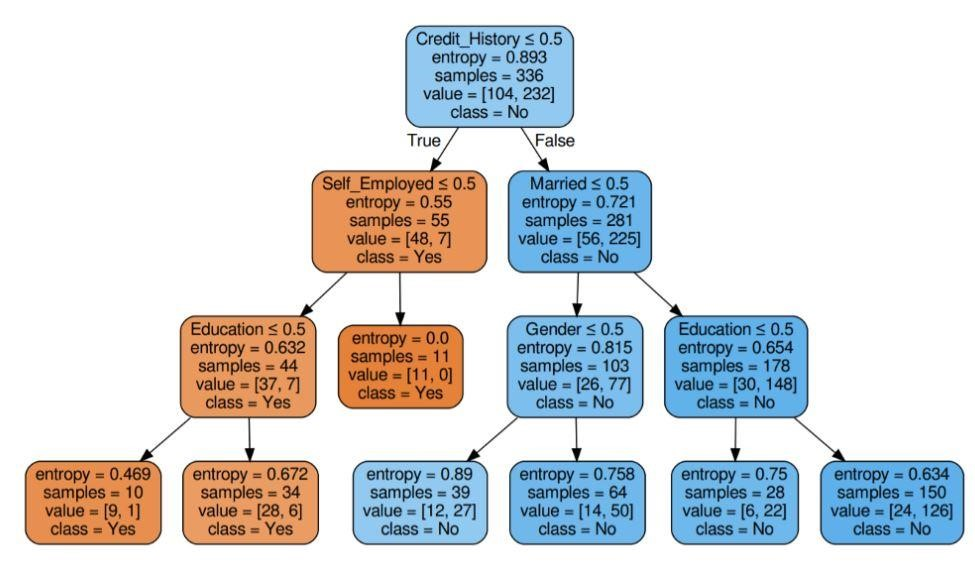Step 6: Check the score of the model

Almost 80% percent accuracy scored.

## List of Applications

Decision trees are mostly used by information experts to carry on an analytical investigation. They might be used extensively for business purposes to analyze or predict difficulties. The flexibility of the decision tree allows them to be used in a different area:

### 1. Healthcare

Decision trees allow the prediction of whether a patient is suffering from a particular disease with conditions of age, weight, sex, etc. Other predictions include deciding the effect of medicine considering factors like composition, period of manufacture, etc.

### 2. Banking sectors

Decision trees help in predicting whether a person is eligible for a loan considering his financial status, salary, family members, etc. It can also identify credit card frauds, loan defaults, etc.

### 3. Educational Sectors

Shortlisting of a student based on his merit score, attendance, etc. can be decided with the help of decision trees.

• The interpretable results of a decision model can be represented to senior management and stakeholders.
• While building a decision tree model, preprocessing of the data, i.e. normalization, scaling, etc. is not required.
• Both types of data- numerical and categorical can be handled by a decision tree which displays its higher efficiency of use over other algorithms.
• Missing value in data doesn’t affect the process of a decision tree thereby making it a flexible algorithm.

## What Next?

If you are interested in gaining hands-on experience in data mining and getting trained by experts in the, you can check out upGrad’s Executive PG Program in Data Science. The course is directed for any age group within 21-45 years of age with minimum eligibility criteria of 50% or equivalent passing marks in graduation. Any working professionals can join this executive PG program certified from IIIT Bangalore.

### What is a Decision Tree in Data Mining?

A decision tree is a way to build models in Data mining. It can be understood as an inverted binary tree. It includes a root node, some branches, and leaf nodes at the end.
Each of the internal nodes in a Decision tree signifies a study on an attribute. Each of the divisions signifies the consequence of that particular study or examination. And finally, each leaf node represents a class tag.
The main objective of building a Decision tree is to create an ideal that can be utilized to foresee the particular class by using judgement procedures on previous data.
We start with the root node, make some relations with the root variable, and make divisions that agree to those values. Based on the base choices, we jump to subsequent nodes.

### What are some of the important nodes used in Decision Trees?

Decision Trees in Data mining have the ability to handle very complicated data. All the decision trees have three vital nodes or portions. Let’s discuss each one of them below.

• Decision Nodes – Each decision node represents a particular decision and is generally displayed with the help of a square.
• Chance Nodes – They usually represent a chance or a confusion and displayed with the help of a circle.
• End Nodes – They are displayed with the help of a triangle and represent a result or a class.

When we connect all these nodes, we get divisions. We can form trees with a variety of difficulties using these nodes and divisions for an infinite number of times.

### What are the advantages of using Decision Trees?

Now that we have understood the working of Decision trees, let’s try to look at a few advantages of using Decision trees in Data mining
1. When we compare them with other methods, Decision trees do not require as much computation for the training of data during pre-processing.
2. Stabilization of information is not involved in Decision trees.
3. Also, they don’t even require scaling of information.
4. Even if some values are omitted in the dataset, this does not interfere in the construction of trees.
5. These models are identical instinctive. They are stress-free for description as well.## 0 replies on “What is Decision Tree in Data Mining? Types, Real World Examples & Applications”